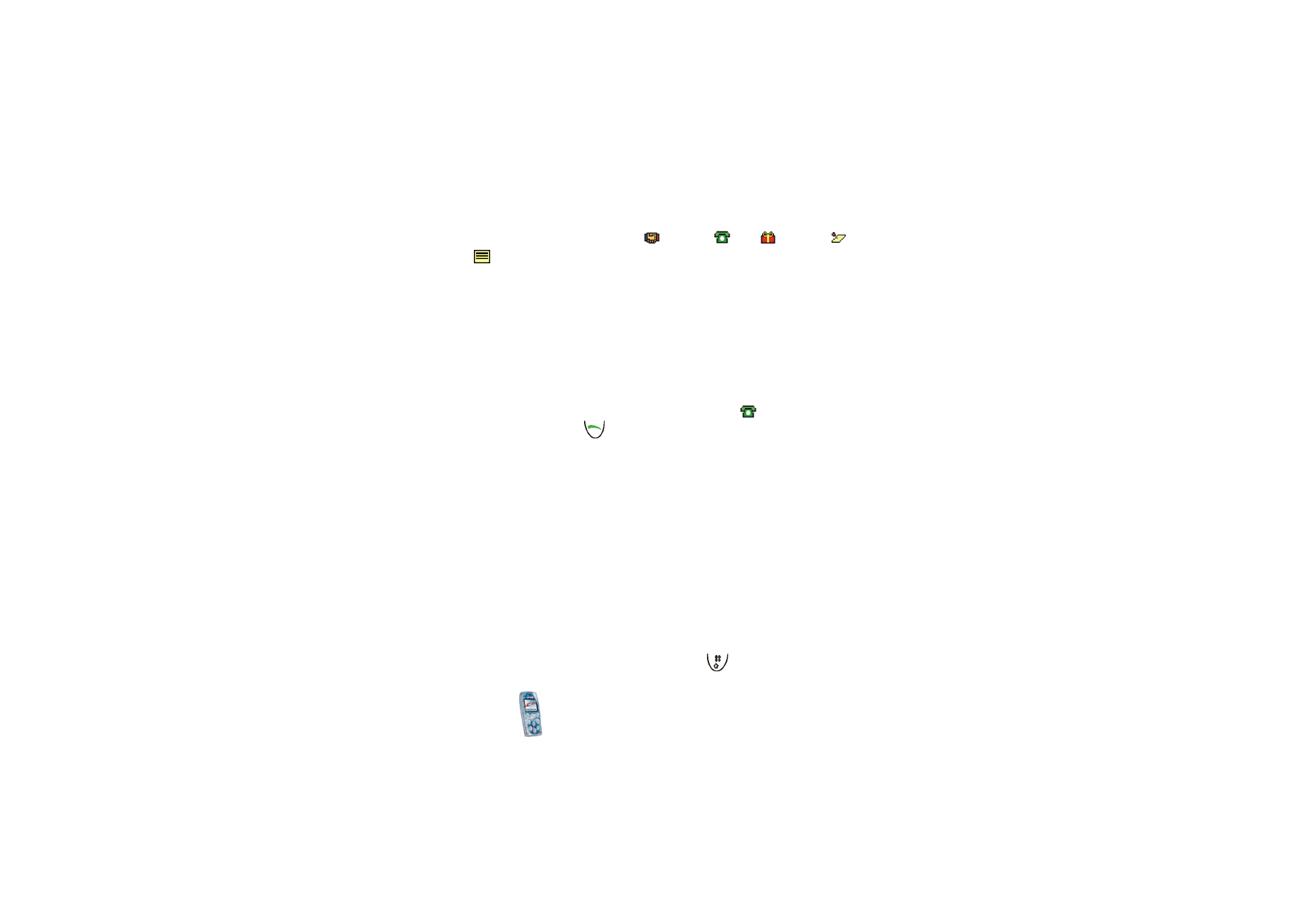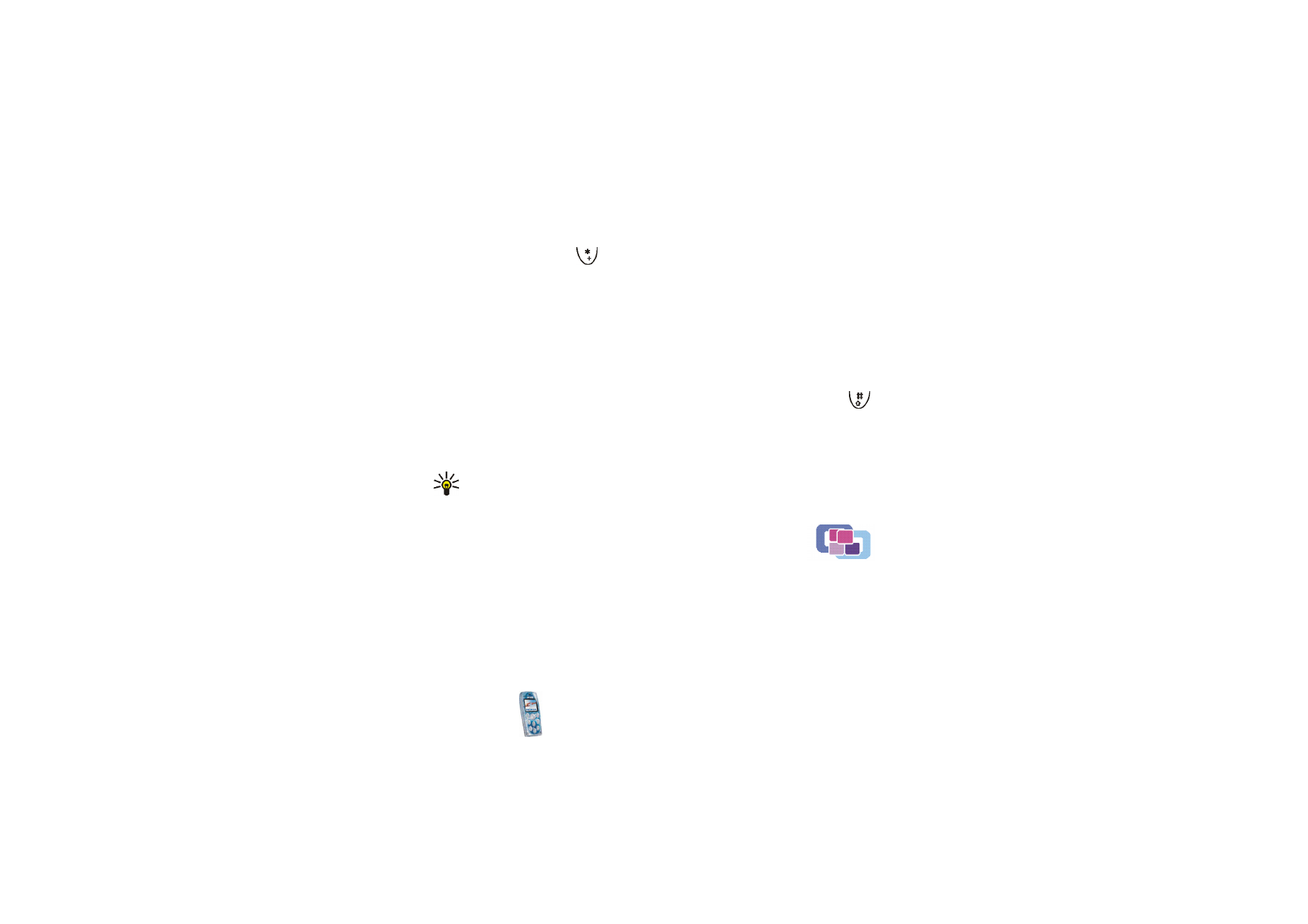## Nokia 3200 - CalculatorCalculator

The phone includes a calculator that can also be used for approximate currency
conversions.

in standby mode, and select

Organiser

and

Calculator

.

This calculator has a limited accuracy and rounding errors may occur, especially in long
divisions.

1. Key in the first number in the calculation. Press

for a decimal point.Men

u

fu

ncti

ons

89

2. Press

Options

and select

,

Subtract

,

Multiply

,

Divide

,

Square

,

Square root

or

Change sign

. OR: Press

once to add, twice to subtract, three times to

multiply or four times to divide.

3. Key in the second number, if needed in the calculation.

4. For a total, press

Options

and select

Equals

.

5. Press and hold

Clear

for a new calculation.